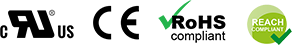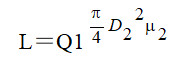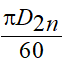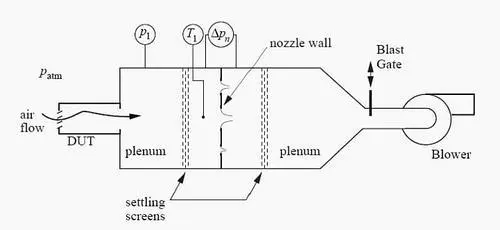﻿ Air Flow And Air Pressure - Dongguan Wanhang Electronic Technology Co., Ltd.
+86-769-8372-8990
johnson@wheecoolingfan.com
en# Air Flow And Air Pressure

Air flow Q, also known as flow rate, is the flow of air passing through the fan outlet section (or air inlet section) in unit time. The unit is generally CFM, namely cubic feet per minute, or CMM, namely cubic meters per minute;

The units used in the dust removal system are m3/s or m3/h.

The air flow of the fan can be measured by the experimental method or can be calculated by calculation, the calculation formula is as followsL -- the air flow of the ventilator, m3/s;

D2- -- Outside diameter of ventilator impeller, m;

Q1 -- Flow coefficient, which is related to fan model;

2 -- the circular velocity of the outer diameter of the impeller, m/s, μ2=(m/s);

N -- ventilator speed, r/min.

Air flow can be adjusted by changing the speed or can be controlled by the gate, pay attention to the maximum speed can not exceed the specified speed.

P refers to the pressure difference generated between the air outlet and the air inlet by the fan. The unit is generally the mm water column, that is, the millimeter water column (similar to the millimeter mercury column that measures atmospheric pressure, but due to the small pressure difference, the unit is generally the water column).

The wind pressure is the difference between the total pressure of the fan inlet and outlet. The unit is mmH2O.

The following formula can be expressed as:

H＝ρH1μ22

H -- the total pressure of the ventilator, mmH2O;

ρ-- Gas density, kg/m3;

H1 -- pressure coefficient, which is related to blade type, is 0.4~0.6 for the backward type and 0.6~0.8 for the radial type;

The forward formula is 0.8~1.1.

The wind pressure can be adjusted by changing the speed of the fan or controlled by a gate.

Air Flow:

You probably have been told that centrifugal fans have higher pressure and lower airflow capabilities than axial fans. However, axial fans have higher airflow and lower pressure capabilities.  What's the truth about different types of fans and blowers in terms of airflow ?

1 CMS = 60 CMM = 3600 CMH

1 CMM = 35.3 CFM

Air Pressure:

1 N/M = 1 Pa (Pascal).

So 1 bar is equal to 10 Pa

1 mm Aq = 1 Kp/M =9.8 Pa

1 in H2 O= 25.4mm H2 O= 25.4mm Aq (Aq = Aqueous)

Both the air flow and the air pressure described by the fan product are the maximum values under the ideal state, namely the air volume under the condition of no pressure difference between the fan inlet and outlet (the maximum air volume), and the difference between the air chamber and the external air pressure under the condition of the fan blowing into the closed air chamber until the air volume is zero (the maximum static pressure).

They are not two independent performance indicators, but mutual constraints, and the relationship between flow rate and pressure is the relationship in fluid mechanics - the amount of wind decreases with the increase of pressure difference,

The measurement of air flow and pressure needs to be carried out with the help of a wind tunnel tester;

Standard of Air Conditioning and Pressure Testing: The Standard of American AMCA-210 (Air Moving and Conditioning Association)At the same time, the choice of the fan, need to consider system damping;

Static pressure is defined as the pressure exerted by a gas on the surface of an object parallel to the flow.

Colloquially speaking: static pressure refers to the pressure to overcome pipe resistance.

Dynamic pressure is defined as the conversion of the kinetic energy required in the flow of gas into the form of pressure.

Colloquially, dynamic pressure is the pressure that drives the forward motion of gas.

Total pressure = static pressure + dynamic pressure

The total pressure is the difference between outlet total pressure and inlet total pressure

Static pressure is the full pressure of the fan to reduce the dynamic pressure at the fan outlet (along with the resistance)

Dynamic pressure is the resistance P generated by itself when the air flows. Dynamic pressure =0.5* density * wind speed squared

P = P(static) + P(dynamic)

1. After two fans with the same model and the same rotation speed are connected in parallel, the highest air flow is about 90% of the air flow of two fans, and the air pressure is equal to the pressure of a single fan.

2. After two fans with the same model and the same speed are connected in series, the air pressure is twice that of a single fan, and the air flow is equal to the air flow of a single fan.

3. The two models are used in parallel at different speeds. The air flow is equal to that of the larger fan, and the air pressure is not superimposed.

4. The two models are different and rotate at different speeds, and the larger one is used in the series before setting. The air pressure is less than that of a single fan, and the air flow is equal to that of the larger one

Related News
Leave us Message